Anúncio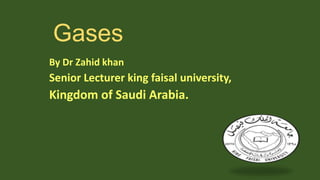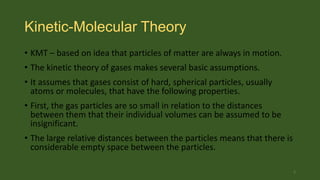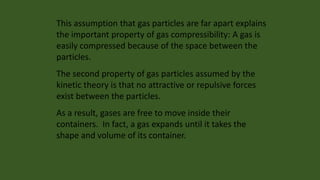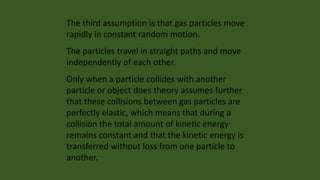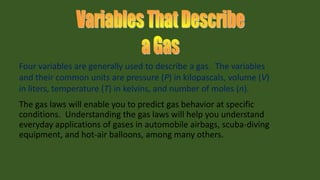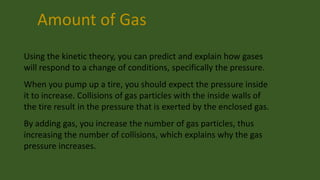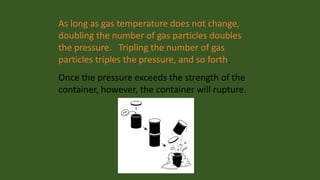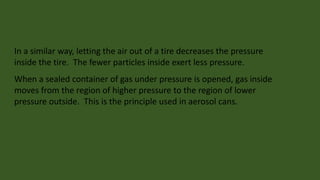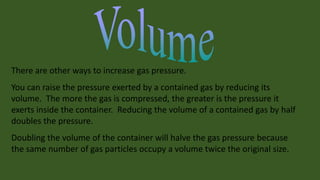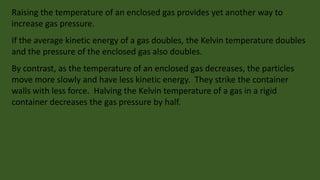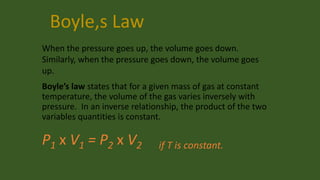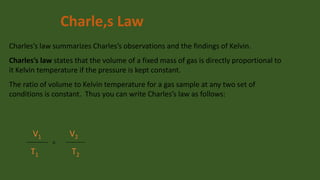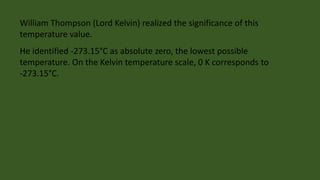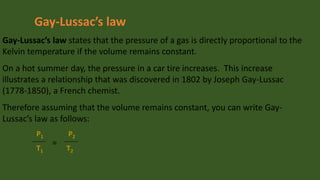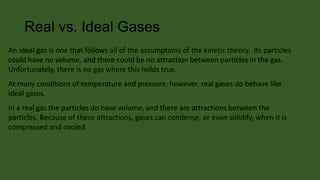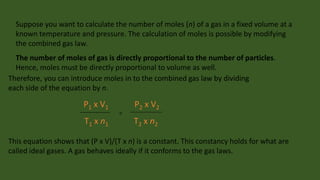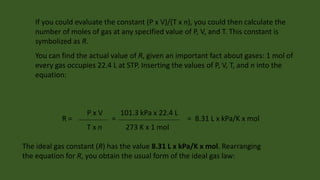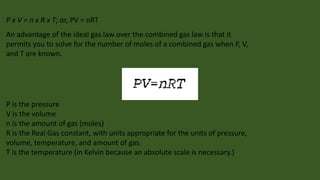1 de 18
Anúncio

### Gases

1. Gases By Dr Zahid khan Senior Lecturer king faisal university, Kingdom of Saudi Arabia.
2. Kinetic-Molecular Theory • KMT – based on idea that particles of matter are always in motion. • The kinetic theory of gases makes several basic assumptions. • It assumes that gases consist of hard, spherical particles, usually atoms or molecules, that have the following properties. • First, the gas particles are so small in relation to the distances between them that their individual volumes can be assumed to be insignificant. • The large relative distances between the particles means that there is considerable empty space between the particles. 2
3. This assumption that gas particles are far apart explains the important property of gas compressibility: A gas is easily compressed because of the space between the particles. The second property of gas particles assumed by the kinetic theory is that no attractive or repulsive forces exist between the particles. As a result, gases are free to move inside their containers. In fact, a gas expands until it takes the shape and volume of its container.
4. The third assumption is that gas particles move rapidly in constant random motion. The particles travel in straight paths and move independently of each other. Only when a particle collides with another particle or object does theory assumes further that these collisions between gas particles are perfectly elastic, which means that during a collision the total amount of kinetic energy remains constant and that the kinetic energy is transferred without loss from one particle to another.
5. Four variables are generally used to describe a gas. The variables and their common units are pressure (P) in kilopascals, volume (V) in liters, temperature (T) in kelvins, and number of moles (n). The gas laws will enable you to predict gas behavior at specific conditions. Understanding the gas laws will help you understand everyday applications of gases in automobile airbags, scuba-diving equipment, and hot-air balloons, among many others.
6. Amount of Gas Using the kinetic theory, you can predict and explain how gases will respond to a change of conditions, specifically the pressure. When you pump up a tire, you should expect the pressure inside it to increase. Collisions of gas particles with the inside walls of the tire result in the pressure that is exerted by the enclosed gas. By adding gas, you increase the number of gas particles, thus increasing the number of collisions, which explains why the gas pressure increases.
7. As long as gas temperature does not change, doubling the number of gas particles doubles the pressure. Tripling the number of gas particles triples the pressure, and so forth. Once the pressure exceeds the strength of the container, however, the container will rupture.
8. In a similar way, letting the air out of a tire decreases the pressure inside the tire. The fewer particles inside exert less pressure. When a sealed container of gas under pressure is opened, gas inside moves from the region of higher pressure to the region of lower pressure outside. This is the principle used in aerosol cans.
9. There are other ways to increase gas pressure. You can raise the pressure exerted by a contained gas by reducing its volume. The more the gas is compressed, the greater is the pressure it exerts inside the container. Reducing the volume of a contained gas by half doubles the pressure. Doubling the volume of the container will halve the gas pressure because the same number of gas particles occupy a volume twice the original size.
10. Raising the temperature of an enclosed gas provides yet another way to increase gas pressure. If the average kinetic energy of a gas doubles, the Kelvin temperature doubles and the pressure of the enclosed gas also doubles. By contrast, as the temperature of an enclosed gas decreases, the particles move more slowly and have less kinetic energy. They strike the container walls with less force. Halving the Kelvin temperature of a gas in a rigid container decreases the gas pressure by half.
11. Boyle,s Law When the pressure goes up, the volume goes down. Similarly, when the pressure goes down, the volume goes up. Boyle’s law states that for a given mass of gas at constant temperature, the volume of the gas varies inversely with pressure. In an inverse relationship, the product of the two variables quantities is constant. P1 x V1 = P 2 x V2 if T is constant.
12. Charle,s Law Charles’s law summarizes Charles’s observations and the findings of Kelvin. Charles’s law states that the volume of a fixed mass of gas is directly proportional to it Kelvin temperature if the pressure is kept constant. The ratio of volume to Kelvin temperature for a gas sample at any two set of conditions is constant. Thus you can write Charles’s law as follows: V1 T1 = V2 T2
13. William Thompson (Lord Kelvin) realized the significance of this temperature value. He identified -273.15°C as absolute zero, the lowest possible temperature. On the Kelvin temperature scale, 0 K corresponds to -273.15°C.
14. Gay-Lussac’s law Gay-Lussac’s law states that the pressure of a gas is directly proportional to the Kelvin temperature if the volume remains constant. On a hot summer day, the pressure in a car tire increases. This increase illustrates a relationship that was discovered in 1802 by Joseph Gay-Lussac (1778-1850), a French chemist. Therefore assuming that the volume remains constant, you can write GayLussac’s law as follows: P1 T1 = P2 T2
15. Real vs. Ideal Gases An ideal gas is one that follows all of the assumptions of the kinetic theory. Its particles could have no volume, and there could be no attraction between particles in the gas. Unfortunately, there is no gas where this holds true. At many conditions of temperature and pressure, however, real gases do behave like ideal gases. In a real gas the particles do have volume, and there are attractions between the particles. Because of these attractions, gases can condense, or even solidify, when it is compressed and cooled.
16. Suppose you want to calculate the number of moles (n) of a gas in a fixed volume at a known temperature and pressure. The calculation of moles is possible by modifying the combined gas law. The number of moles of gas is directly proportional to the number of particles. Hence, moles must be directly proportional to volume as well. Therefore, you can introduce moles in to the combined gas law by dividing each side of the equation by n. P1 x V1 T 1 x n1 = P2 x V2 T 2 x n2 This equation shows that (P x V)/(T x n) is a constant. This constancy holds for what are called ideal gases. A gas behaves ideally if it conforms to the gas laws.
17. If you could evaluate the constant (P x V)/(T x n), you could then calculate the number of moles of gas at any specified value of P, V, and T. This constant is symbolized as R. You can find the actual value of R, given an important fact about gases: 1 mol of every gas occupies 22.4 L at STP. Inserting the values of P, V, T, and n into the equation: R= PxV Txn = 101.3 kPa x 22.4 L = 8.31 L x kPa/K x mol 273 K x 1 mol The ideal gas constant (R) has the value 8.31 L x kPa/K x mol. Rearranging the equation for R, you obtain the usual form of the ideal gas law:
18. P x V = n x R x T; or, PV = nRT An advantage of the ideal gas law over the combined gas law is that it permits you to solve for the number of moles of a combined gas when P, V, and T are known. P is the pressure V is the volume n is the amount of gas (moles) R is the Real Gas constant, with units appropriate for the units of pressure, volume, temperature, and amount of gas. T is the temperature (in Kelvin because an absolute scale is necessary.)
Anúncio